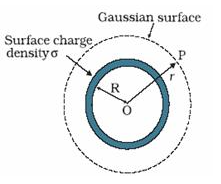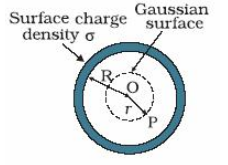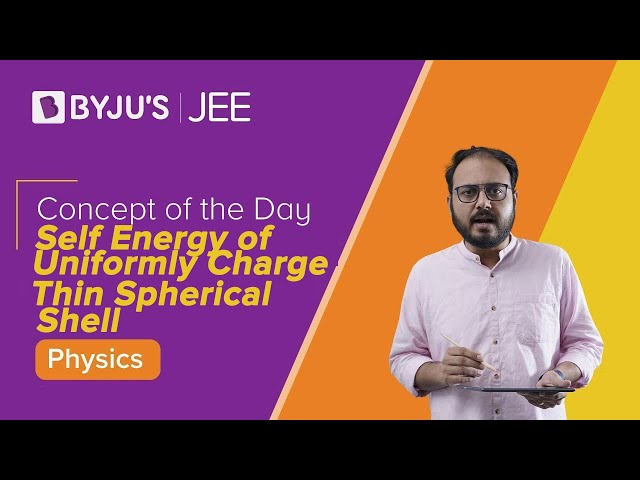Checkout JEE MAINS 2022 Question Paper Analysis : Checkout JEE MAINS 2022 Question Paper Analysis :

# Electric Field Intensity Due To A Thin Uniformly Charged Spherical Shell

Force F applied on the unit positive electric charge q at a point describes the electric field. The formula to find the electric field is E=F/q. The direction of the force applied on a negative charge is opposite to that exerted on a positive charge. The electric field is a vector quantity that has both direction and magnitude. The electric field is represented by field lines or lines of force.

In this article, let us learn in detail about electric field intensity due to a uniformly charged spherical shell.

## Electric Field Intensity Due To A Thin Uniformly Charged Spherical Shell

In this derivation, we have three cases

1. The electric field outside the shell
2. The electric field inside the shell
3. The electric field at the surface of the shell

Electric Field Outside The Shell

Consider a point P, placed outside the spherical shell. Here, OP=r. As shown in the figure below, the Gaussian surface as a sphere is assumed to have radius r. The electric field intensity,

$$\begin{array}{l}\bar{E}\end{array}$$
is said to be the same at every point of a Gaussian surface directed outwards.In the above mentioned Gausssian surface, since the electric field is directed outwards (as in unit vector

$$\begin{array}{l}\hat{n}\end{array}$$

When,

$$\begin{array}{l}\Theta = 0^{0}\end{array}$$
$$\begin{array}{l}\oint_{s}^{}\bar{E}.\bar{ds} = \oint_{s}^{}\bar{E}.\hat{n}.{ds}= \frac{q}{\epsilon _{0}}\end{array}$$

Or

$$\begin{array}{l}{E} \oint {dS} = \frac{q}{\epsilon _{0}}\end{array}$$
$$\begin{array}{l}{E} (4\pi r^{2}) = \frac{q}{\epsilon _{0}}\end{array}$$

Or

$$\begin{array}{l}{E} = \frac{q}{4\pi r^{2}\epsilon _{0}}\end{array}$$

We can note that electric intensity at any point outside the spherical shell looks like the complete charge is concentrated at the midpoint of the shell.

Electric Field Inside The ShellConsider the point P placed inside the shell. As shown in the figure above, the Gaussian surface is said to have radius r. The Gaussian surface contains no charge inside it.

Hence, q=0

Therefore,

E = 0

Hence, we can conclude that the field inside the spherical shell is always zero.

Electric Field At The Surface Of The Shell

Consider, r = R.

Hence,

$$\begin{array}{l}{E} = \frac{q}{4\pi R^{2}\epsilon _{0}}\end{array}$$

Consider the charge density on the shell to be \sigma Cm^{-2}

$$\begin{array}{l}q = 4\pi R^{2}. \sigma\end{array}$$
$$\begin{array}{l}q = \frac{4\pi R^{2}. \sigma }{4\pi R^{2}. \epsilon _{0}} = \frac{\sigma }{\epsilon _{0}}\end{array}$$

 Related links Relative motion protection against earthquake Lenz law Radioactivity – alpha decay

Stay tuned with BYJU’S for more such interesting derivations in physics, chemistry and maths in an engaging way with video explanations.

## Frequently Asked Questions on Electric Field

Zero.

### 2. What is the electric field at the surface of the spherical shell?

$$\begin{array}{l}q = \frac{4\pi R^{2}. \sigma }{4\pi R^{2}. \epsilon _{0}} = \frac{\sigma }{\epsilon _{0}}\end{array}$$

### 3. What is the electric field outside the surface of the spherical shell?

$$\begin{array}{l}{E} = \frac{q}{4\pi r^{2}\epsilon _{0}}\end{array}$$

E=F/q.

### 5. Electric field is a vector or scalar quantity?

Electric field is a vector quantity.

The video explains how to obtain the self-energy of a uniformly charged thin spherical shell.